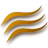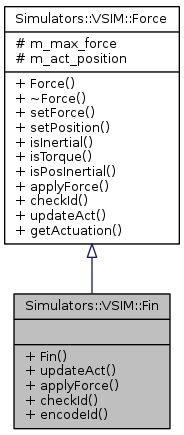DUNE: Uniform Navigational Environment  2016.05.0
Simulators::VSIM::Fin Class Reference

## Detailed Description

Fin control surfaces class.

## Public Member Functions

Fin (unsigned int finid, double=0, double=0)

void updateAct (double value)

void applyForce (double speed, double forces)

bool checkId (unsigned int testid)Public Member Functions inherited from Simulators::VSIM::Force
Force (void)

virtual ~Force (void)

void setForce (double, double, double, bool, bool)

void setPosition (double, double, double, bool)

bool isInertial (void)

bool isTorque (void)

bool isPosInertial (void)

virtual double getActuation (void)

## Static Public Member Functions

static int encodeId (int id)Protected Attributes inherited from Simulators::VSIM::Force
double m_max_force 

double m_act_position 

## Constructor & Destructor Documentation

 Simulators::VSIM::Fin::Fin ( unsigned int finid, double coef = `0`, double pos = `0` )

Constructor.

Parameters
 [in] finid fin id. [in] coef fin coeficients. [in] pos fin position.

## Member Function Documentation

 void Simulators::VSIM::Fin::applyForce ( double speed, double forces )
virtual

Apply fin's force.

Parameters
 [in] speed speed reference. [out] forces forces to be applied by the fin.

Reimplemented from Simulators::VSIM::Force.

 bool Simulators::VSIM::Fin::checkId ( unsigned int testid )
virtual

Check fin's id.

Returns
true if id matches, false otherwise.

Reimplemented from Simulators::VSIM::Force.

 static int Simulators::VSIM::Fin::encodeId ( int id )
inlinestatic

Fin id encoding.

Referenced by Simulators::VSIM::UUV::updateFin().

 void Simulators::VSIM::Fin::updateAct ( double value )
virtual

Update fin's actuation.

Parameters
 [in] value fin actuation.

Reimplemented from Simulators::VSIM::Force.

Collaboration diagram for Simulators::VSIM::Fin: